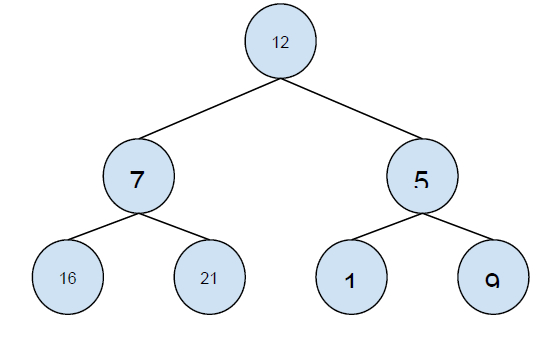# Preorder from Inorder and Postorder traversals in C++

In this problem, we are given the inorder and postorder traversal of a binary tree. Our task is to print the postorder traversal of the tree.

Let’s take an example to understand the problem

Input:inorder: 16 7 21 12 1 5 9
postorder: 16 21 7 1 9 5 12
Output: preorder: 12 7 16 21 5 1 9
Explanation: the binary tree is :To solve this problem, a simple solution could be creating a tree using the given traversals and then finding the preorder traversal of the tree. But this method will be more complex for the system.

A more effective solution to solve the problem is using the stack data structure. Let’s see each node of the tree. The root of the tree is the last item in postorder traversal. Now we have to separate the left and right subtree of the binary tree. Since we know the root node of the tree. In the postorder traversal, all elements before the root node are of left subtree and after the root are of right subtree.

Like this, we will find all elements and store the nodes in the stack and the print elements of the stack which gives the preorder traversal.

The implementation of our solution in Java

## Example

Live Demo

import java.util.Stack;
public class Main {
static int postIndex;
void preOrder(int[] in, int[] post, int inStrt, int inEnd, Stack<Integer> preorder) {
if (inStrt > inEnd)
return;
int val = post[postIndex];
int inIndex = searchValue(in, val);
postIndex--;
preOrder(in, post, inIndex + 1, inEnd, preorder);
preOrder(in, post, inStrt, inIndex - 1, preorder);
preorder.push(val);
}
void printPreOrderTraversal(int[] in, int[] post) {
int len = in.length;
postIndex = len - 1;
Stack<Integer> preorder = new Stack<Integer>();
preOrder(in, post, 0, len - 1, preorder);
while (preorder.empty() == false)
System.out.print(preorder.pop()+" ");
}
int searchValue(int[] in, int data){
int i = 0;
for (i = 0; i < in.length; i++)
if (in[i] == data)
return i;
return i;
}
public static void main(String ars[]){
int in[] = { 4, 10, 12, 15, 18, 22, 24, 25, 31, 35, 44, 50, 66, 70, 90 };
int post[] = { 4, 12, 10, 18, 24, 22, 15, 31, 44, 35, 66, 90, 70, 50, 25 };
Main tree = new Main();
System.out.println("Preorder Traversal of the tree is: ");
tree.printPreOrderTraversal(in, post);
}
}

## Output

Preorder Traversal of the tree is:
25 15 10 4 12 22 18 24 50 35 31 44 70 66 90